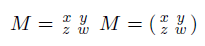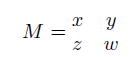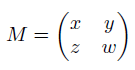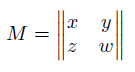## Monday, May 18, 2009

### How to create a matrix in LaTeX

We can create a matrix in LaTeX using the array environment, or simplematrix, matrix, pmatrix, bmatrix, vmatrix, and Vmatrix environments via amsmath package. This article provides some examples on how to create a matrix in LaTeX.

### Creating a matrix with array

Here are some examples.
1. unbracketed matrix$M = \begin{array}{cc} x & y \\ z & w \end{array}$
2. matrix surrounded by square brackets$M = \left[ {\begin{array}{cc} x & y \\ z & w \end{array} } \right]$
3. matrix surrounded by parentheses$M = \left( {\begin{array}{cc} x & y \\ z & w \end{array} } \right)$
4. matrix surrounded by single vertical lines$M = \left| {\begin{array}{cc} x & y \\ z & w \end{array} } \right|$

### Using amsmath package

Call \usepackage{amsmath} in the preamble, after documentclass{}.

The amsmath package environment for matrix:
1. smallmatrix: inline matrix$M = \begin{smallmatrix} x & y \\ z & w \end{smallmatrix}$

$M = \left( \begin{smallmatrix} x & y \\ z & w \end{smallmatrix}\right)$
2. matrix: unbracketed matrix$M = \begin{matrix} x & y \\ z & w \end{matrix}$

3. pmatrix: matrix surrounded by parentheses$M = \begin{pmatrix} x & y \\ z & w \end{pmatrix}$
4. bmatrix: matrix surrounded by square brackets$M = \begin{bmatrix} x & y \\ z & w \end{bmatrix}$
5. vmatrix: matrix surrounded by single vertical lines$M = \begin{vmatrix} x & y \\ z & w \end{vmatrix}$
6. Vmatrix: matrix surrounded by double vertical lines$M = \begin{Vmatrix} x & y \\ z & w \end{Vmatrix}$